# Indefinite Integrals

## Calculus 1 / Ab### 9200 Practice Problems

07:43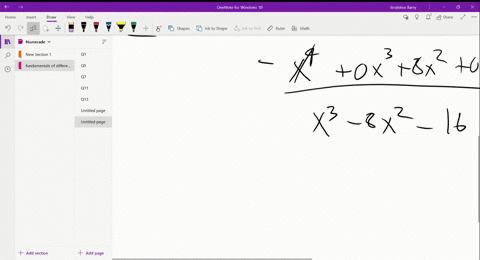Calculus: Early Transcendental Functions

In exercises find the partial lractions decomposition.
$$\frac{x^{4}+x^{3}}{\left(x^{2}+4\right)^{2}}$$

Integration Techniques
Integration of Rational Functions using Partial Fractions05:13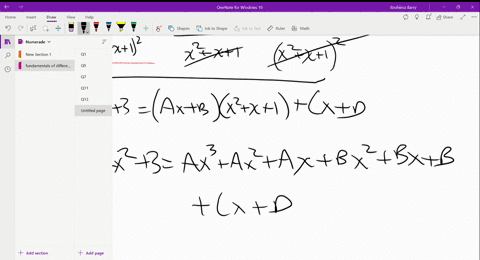Calculus: Early Transcendental Functions

In exercises find the partial lractions decomposition.
$$\frac{4 x^{2}+3}{\left(x^{2}+x+1\right)^{2}}$$

Integration Techniques
Integration of Rational Functions using Partial Fractions03:48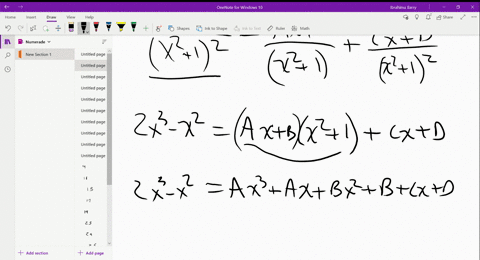Calculus: Early Transcendental Functions

In exercises find the partial lractions decomposition.
$$\frac{2 x^{3}-x^{2}}{\left(x^{2}+1\right)^{2}}$$

Integration Techniques
Integration of Rational Functions using Partial Fractions03:24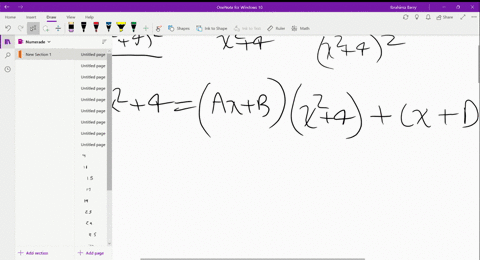Calculus: Early Transcendental Functions

In exercises find the partial lractions decomposition.
$$\frac{2 x^{2}+4}{\left(x^{2}+4\right)^{2}}$$

Integration Techniques
Integration of Rational Functions using Partial Fractions07:22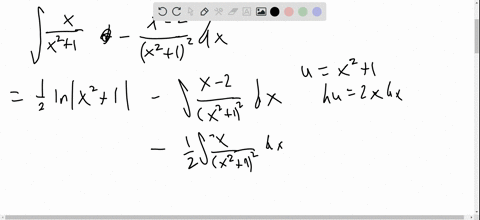Calculus: Early Transcendental Functions

In exercises find the partial lractions decomposition.
$$\frac{x^{3}+2}{\left(x^{2}+1\right)^{2}}$$

Integration Techniques
Integration of Rational Functions using Partial Fractions01:44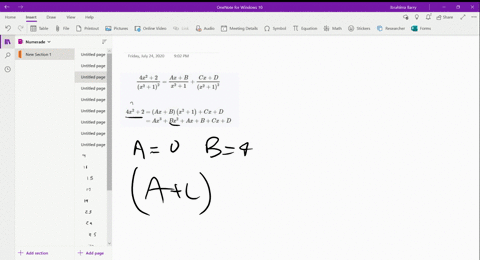Calculus: Early Transcendental Functions

In this exercise, we find the partial fractions decomposition of $\frac{4 x^{2}+2}{\left(x^{2}+1\right)^{2}} .$ Consistent with the form for repeated linear factors, the form for the decomposition is $\frac{A x+B}{x^{2}+1}+\frac{C x+D}{\left(x^{2}+1\right)^{2}}$ We set
$$\frac{4 x^{2}+2}{\left(x^{2}+1\right)^{2}}=\frac{A x+B}{x^{2}+1}+\frac{C x+D}{\left(x^{2}+1\right)^{2}}$$
Multiplying through by $\left(x^{2}+1\right)^{2},$ we get
\begin{aligned} 4 x^{2}+2 &=(A x+B)\left(x^{2}+1\right)+C x+D \\ &=A x^{3}+B x^{2}+A x+B+C x+D \end{aligned}
As in example $4.5,$ we match up coefficients of like powers of $x$. For $x^{3}$, we have $0=A$. For $x^{2}$, we have $4=B$. Match the coefficients of $x$ and the constants to finish the decomposition.

Integration Techniques
Integration of Rational Functions using Partial Fractions01:58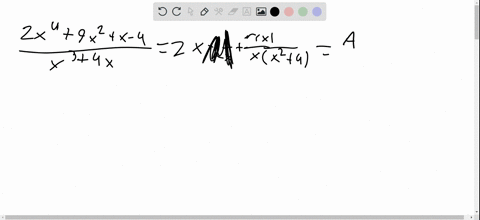Calculus: Early Transcendental Functions

In exercises find the partial fractions decomposition and an antiderivative. If you have a CAS available, use it to check your answer.
$$\frac{2 x^{4}+9 x^{2}+x-4}{x^{3}+4 x}$$

Integration Techniques
Integration of Rational Functions using Partial Fractions01:59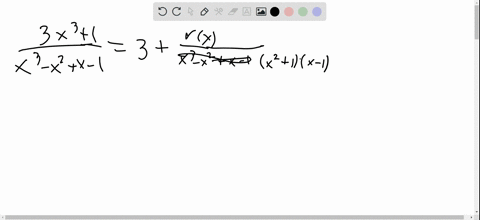Calculus: Early Transcendental Functions

In exercises find the partial fractions decomposition and an antiderivative. If you have a CAS available, use it to check your answer.
$$\frac{3 x^{3}+1}{x^{3}-x^{2}+x-1}$$

Integration Techniques
Integration of Rational Functions using Partial Fractions04:24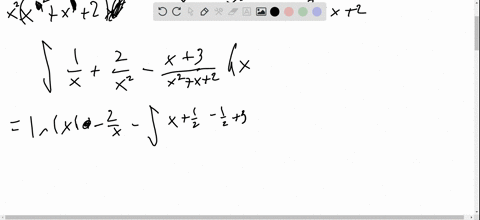Calculus: Early Transcendental Functions

In exercises find the partial fractions decomposition and an antiderivative. If you have a CAS available, use it to check your answer.
$$\frac{4 x+4}{x^{4}+x^{3}+2 x^{2}}$$

Integration Techniques
Integration of Rational Functions using Partial Fractions04:03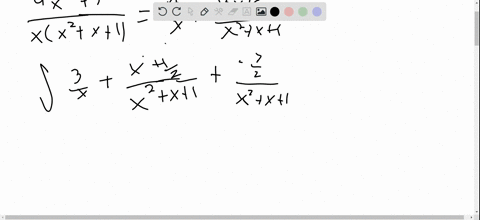Calculus: Early Transcendental Functions

In exercises find the partial fractions decomposition and an antiderivative. If you have a CAS available, use it to check your answer.
$$\frac{4 x^{2}+3}{x^{3}+x^{2}+x}$$

Integration Techniques
Integration of Rational Functions using Partial Fractions05:56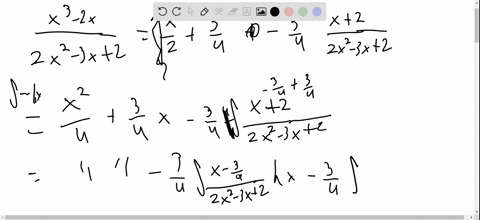Calculus: Early Transcendental Functions

In exercises find the partial fractions decomposition and an antiderivative. If you have a CAS available, use it to check your answer.
$$\frac{x^{3}-2 x}{2 x^{2}-3 x+2}$$

Integration Techniques
Integration of Rational Functions using Partial Fractions09:04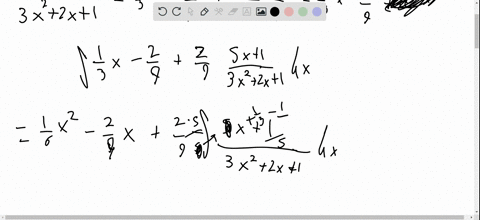Calculus: Early Transcendental Functions

In exercises find the partial fractions decomposition and an antiderivative. If you have a CAS available, use it to check your answer.
$$\frac{x^{3}+x}{3 x^{2}+2 x+1}$$

Integration Techniques
Integration of Rational Functions using Partial Fractions1 2 3 4 5 ... 767